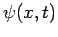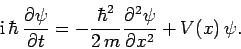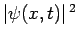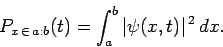Next: Normalization of the Wavefunction Up: Fundamentals of Quantum Mechanics Previous: Introduction

# Schrödinger's Equation

Consider a dynamical system consisting of a single non-relativistic particle of massmoving along the-axis in some real potential. In quantum mechanics, the instantaneous state of the system is represented by a complex wavefunction. This wavefunction evolves in time according to Schrödinger's equation:(137)

The wavefunction is interpreted as follows:is the probability density of a measurement of the particle's displacement yielding the value. Thus, the probability of a measurement of the displacement giving a result betweenand(where) is(138)

Note that this quantity is real and positive definite.

Richard Fitzpatrick 2010-07-20